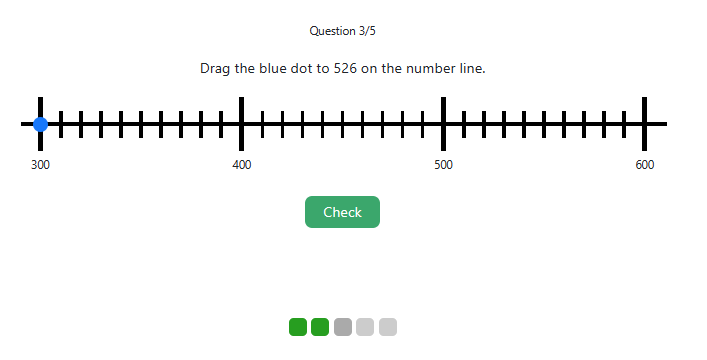MM Practice
×
Multiplication
Division
Place Value
Fractions & Decimals
Measurement
Statistics & Probability
Pre-Algebra
Money
Kindergarten
Geometry

# Plot numbers and integers on the number lineOnline practice for grades 1-3 and 6

In this online activity, you drag a dot to position (plot) the given number on a number line, practicing place value and estimation.

In the "normal" mode, you will see plenty of tick marks on the number line. In the "Estimation" mode, there are only a few tick marks, and you have to estimate where to place the dot on the number line.

Options include 2-digit, 3-digit, and/or 4-digit numbers; positive and/or negative numbers (integers), and how many questions you get.

• For 1st grade and Kindergarten, choose the 2-digit numbers.
• For 2nd grade, choose the 3-digit numbers.
• For 3rd grade, choose the 4-digit numbers.
• For 6th grade, choose the negative numbers and all the number ranges.

You can generate a quick link with specific options and send that link to your students to practice in a specific way.

Screenshot:Question 1/5